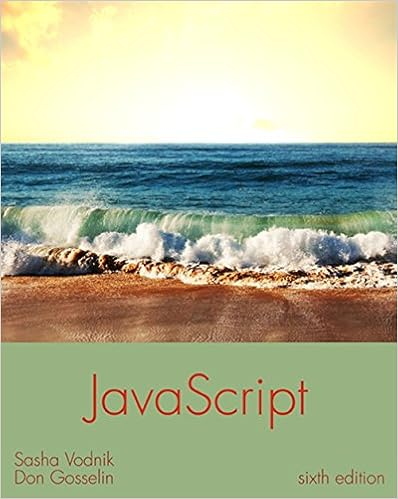# What is difference between syntactic and run-time error? 2...

• Homework Help
• 3

This preview shows page 1 - 2 out of 3 pages.

##### We have textbook solutions for you!
The document you are viewing contains questions related to this textbook.The document you are viewing contains questions related to this textbook.
Chapter 4 / Exercise 1
JavaScript: The Web Warrior Series
VodnikExpert Verified
CS 135 — Problem Set 1 Winter 2017 [Theory] 1. What is difference between syntactic and run-time error? 2. Why this expression does not produce run-time error? (and true (= 4 5) (/ 0 0)) 3. Spot errors(if there is any) in the following code and state what type error it is: (define (f x x)(+ x x)) (define (z f x)(* f x)) (define (k l m)(/ k l)) (define (foo one two)(/ (- one one) (- two two))) (define (CONDITIONS arg1)(cond[false true] . [(or false false) arg1] . [(and false true) ’End])) 4. Why constants are useful? 5. What is difference between check-within and check-expect and where each used? 6. Name and describe 5 parts of Design Recipe. 7. Why using symbol=? comparison between symbols is preferred over the use equal ? ? 8. What is difference between white-box and black-box testing? 9. What is predicate ? 10. What are the good test cases for the following function? . (define (whithin-range? x) . (cond[( < x 10) true] . [( < = x 20) false] . [else true])) [Practice] 1. Create a function interval 1? which takes as input point x and returns true if x is in interval [4, 8], and false otherwise. Do not use cond.
##### We have textbook solutions for you!
The document you are viewing contains questions related to this textbook.The document you are viewing contains questions related to this textbook.
Chapter 4 / Exercise 1
JavaScript: The Web Warrior Series
VodnikExpert Verified
•••